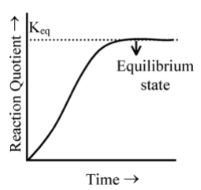# Reaction Quotient Prediction Of Direction Of Reaction

## Reaction Quotient Prediction Of Direction Of Reaction

Suppose a gaseous mixture consists of 0.02 M CO, 0.02 M H2, 0.001 M CH4 and 0.001 M H2O. If the mixture is passed over a catalyst at 1200 K, the following reaction occurs

CO(g) + 3H2(g)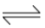CH4(g) + H2O(g); Kc = 3.0 ×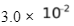Would the reaction start from the right or from the left to attain equilibrium ? That is, would the mixture form more CH4 and H2O in going toward equilibrium or would it form more CO and H2 ?

To answer this problem, we are introducing a new term called reaction quotient, Qc. Reaction quotient is an expression that has the same form as the equilibrium constant expression, but whose concentration values are not necessarily those at equilibrium, it can be at any instant of time. To predict the direction, we substitute the concentrations of substances into the reaction quotient and compare its value to Kc.

Qc =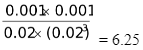As the value of Qc is more than Kc, this value will tend to approach the value of Kc. This means Qc has to decrease which is possible only when products are converted to reactants i.e. reaction starts proceeding in the reverse direction to attain equilibrium.

For the general reaction : aA + bBcC + dD

Qc =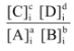where the subscript i indicates concentrations of species at a particular instant, i.

Then,if Qc > Kc, the reaction will go to the left.

If Qc < Kc, the reaction will go to the right.

If Qc = Kc, the reaction mixture is at equilibrium.

 It is clear from the figure that as the reaction proceeds, the value of Q increases as the concentrations of the products increase and the concentrations of the reactants simultaneously decreases. When the reaction reaches equilibrium, the value of the reaction quotient no longer changes as it becomes equal to be value of equilibrium constant.